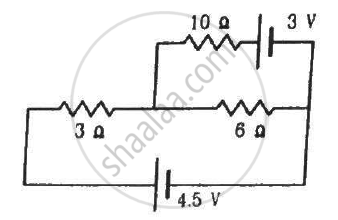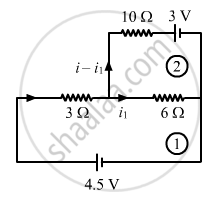Advertisement Remove all ads

# Find the Current Through the 10 ω Resistor Shown in the Figure. - Physics

Sum

Find the current through the 10 Ω resistor shown in the figure.Advertisement Remove all ads

#### SolutionApplying KVL in loop 1, we get:-

3i + 6i1 = 4.5 ...............(1)

Applying KVL in loop 2, we get:-

$\left( i - i_1 \right)10 + 3 - 6 i_1 = 0$

$10i - 16 i_1 = - 3 ..............(2)$

Multiplying equation (1) by 10 and (2) by 3 and then, subtracting (2) from (1), we get:-

$- 108 i_1 = - 54$

$\Rightarrow i_1 = \frac{54}{108} = \frac{1}{2} = 0 . 5$

Substituting the value of i1 in (1), we get:-

$3i + 6 \times \frac{1}{2} - 4 . 5 = 0$

$3i - 1 . 5 = 0$

$\Rightarrow i = \frac{1 . 5}{3} = 0 . 5$

So, current flowing through the 10 Ω resistor = i - i1 = 0.5 - 0.5 = 0 A

Is there an error in this question or solution?
Advertisement Remove all ads

#### APPEARS IN

HC Verma Class 11, Class 12 Concepts of Physics Vol. 2
Chapter 10 Electric Current in Conductors
Q 38 | Page 200
Advertisement Remove all ads

#### Video TutorialsVIEW ALL 

Advertisement Remove all ads
Share
Notifications

View all notifications

Forgot password?